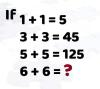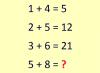BRAIN TEASERSBrain Teasers User Profile

# Mushfiqur Siam

rank
619
points
2
See full ranking list
short ranking list
 617 Dilip Lalwani 2 618 carol lim 2 619 Mushfiqur Siam 2 620 Константин Суворов 2 621 Lucas Tsui 2
 Mathematical Puzzle: IF 1+1=... Mathematical Puzzle: IF 1+1=5, 3+3=45 and 5+5=125 THEN 6+6=?Calculate 5+8 If 1+4=5, 2+5=12 and 3+6=21 then 5+8=?